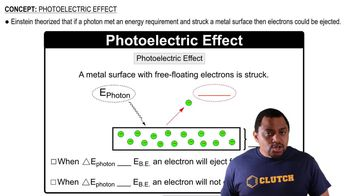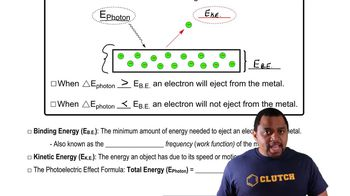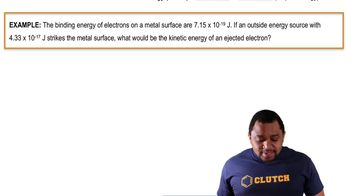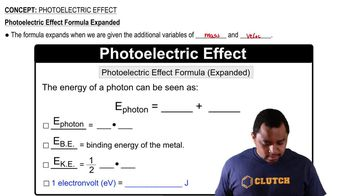Start typing, then use the up and down arrows to select an option from the list.
5:25 minutes
Problem 132
Textbook Question

# The amount of energy necessary to remove an electron from an atom is a quantity called the ionization energy, Ei. This energy can be measured by a technique called photoelectron spectroscopy, in which light of wavelength l is directed at an atom, causing an electron to be ejected. The kinetic energy of the ejected electron (Ek) is measured by determining its veloc-ity, v (Ek = mv2/2), and Ei is then calculated using the conservation of energy principle. That is, the energy of the incident light equals Ei plus Ek. What is the ionization energy of selenium atoms in kilojoules per mole if light with l = 48.2 nm produces electrons with a velocity of 2.371 * 106 m/s? The mass, m, of an electron is 9.109 * 10-31 kg.Verified Solution
This video solution was recommended by our tutors as helpful for the problem above.
371views

### Watch next

Master Photoelectric Effect with a bite sized video explanation from Jules Bruno

Start learning01:2601:1101:2201:1801:52